# High School Chemistry : Using Moles

## Example Questions

### Example Question #3 : High School Chemistry

Consider the following four samples: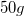of potassiumof lithiumof magnesiumof chlorine gas

Which of the given samples contains the most atoms?

Lithium

They all have the same number of atoms

Potassium

Chlorine

Lithium

Explanation:

It is important to note that the mass of a sample does not tell you the amount of atoms in the sample. The number of atoms in a sample is dependent on the number moles in a sample, given by Avogadro's number. Here is the number of moles for each sample: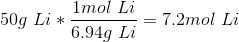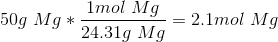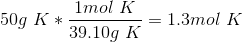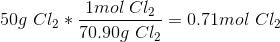Remember that chlorine is a diatomic mass, so each molecules contains two atoms. This doubles the molar mass for the conversion.

The sample with the greatest number of moles will also contain the most atoms. In this case, the sample of lithium results in the largest number of moles and, thus, the greatest number of atoms.

### Example Question #8 : Units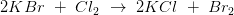Consider the reaction above. If you start with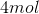of potassium bromide, how many moles of bromine are produced? How many molecules is this equal to?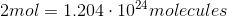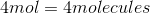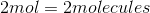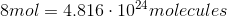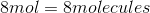Explanation:

In the chemical equation, the ratio of potassium bromide to bromine is 2:1, so for every 2 moles of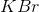, 1 mole of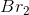is produced. Therefore, if we start with 4 moles of, we get 2 moles of. The number of molecules is equal to the number of moles times Avogadro's Number. Since we've determined the number of moles to be 2, the number of molecules is: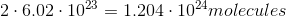### Example Question #1 : Measurements

Convert the following amount from grams (g) to moles (m)

How many moles is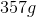of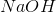?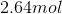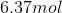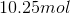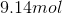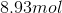Explanation:

Use the periodic table to calculate the molecular weight of sodium hydroxide.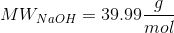Next, use dimensional analysis to find the number of moles.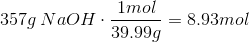### Example Question #1 : Units

How many moles of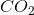are present in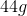of the substance?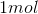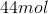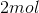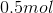The molecular weight of carbon dioxide is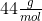, meaning inof, there areof the substance.